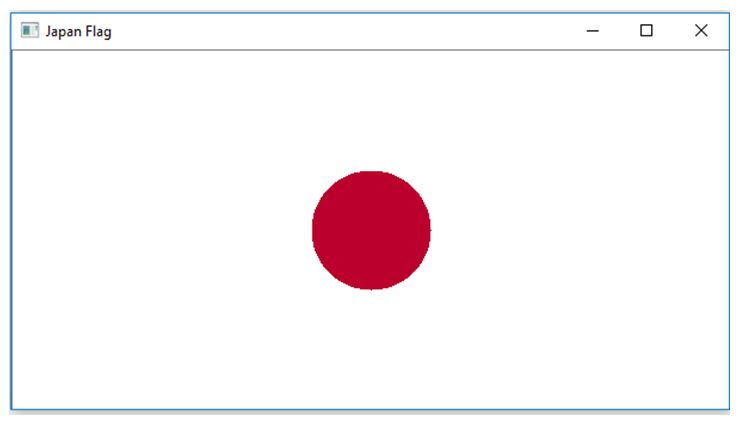# Drawing flag of Japan | Image processing in Python

This is an example of image processing in python – here we are going to learn how to draw a flag of Japan in python?
Submitted by Ankit Rai, on June 11, 2019

Read basics of the drawing/image processing in python: Drawing flag of Thailand

The national flag of Japan is a rectangular white banner bearing a crimson-red disc at its center. This flag is officially called Nisshōki but is more commonly known in Japan as Hinomaru. It embodies the country's sobriquet: Land of the Rising Sun.

Steps:

First, we make a matrix of dimensions 300 X 600 X 3. Where the number of pixels of rows is 300, the number of pixels of columns is 600 and 3 represent the number of dimensions of the color coding in BGR format.

• Paint the complete image with white color. BGR code for White is (255,255,255).
• Apply loop on rows and columns and implement the equation of the circle such that we get a circle in the center of the flag and color it crimson glory using RGB format.

Equation of circle:

```    ((x-h)^2 - (y-k)^2)=r^2
```

Where (h, k) are the centres, (x, y) are co-ordinates of x-axis and y-axis and r is the radius of the circle.

bgrcode for crimson glory color is (45, 0, 188).

## Python code to draw flag of Japan

```# import numpy library as np
import numpy as np

# import open-cv library
import cv2

# import sqrt function from the math module
from math import sqrt

# here image is of class 'uint8', the range of values
# that each colour component can have is [0 - 255]

# create a zero matrix of order 300x600 of 3-dimensions
flag = np.zeros((300, 600, 3),np.uint8)

# take coordinate of the circle
center_x, center_y = 150, 300

# take radius of the circle

# fill whole pixels of dimensions
# with White color
flag[:, :, :] = 255;

# Draw a circle with crimson glory color

# loop for rows i.e. for x-axis
for i in range(101,201) :

# loop for columns i.e. for y-axis
for j in range(251, 351) :

#applying the equation of circle to make the circle in the center.
distance = sqrt((center_x - i)**2 + (center_y - j)**2)

# fill the circle with crimson glory
# color using RGB color representation.
flag[i, j, 0] = 45
flag[i, j, 1] = 0
flag[i, j, 2] = 188

# Show the image formed
cv2.imshow("Japan Flag",flag);
```

OutputPreparation